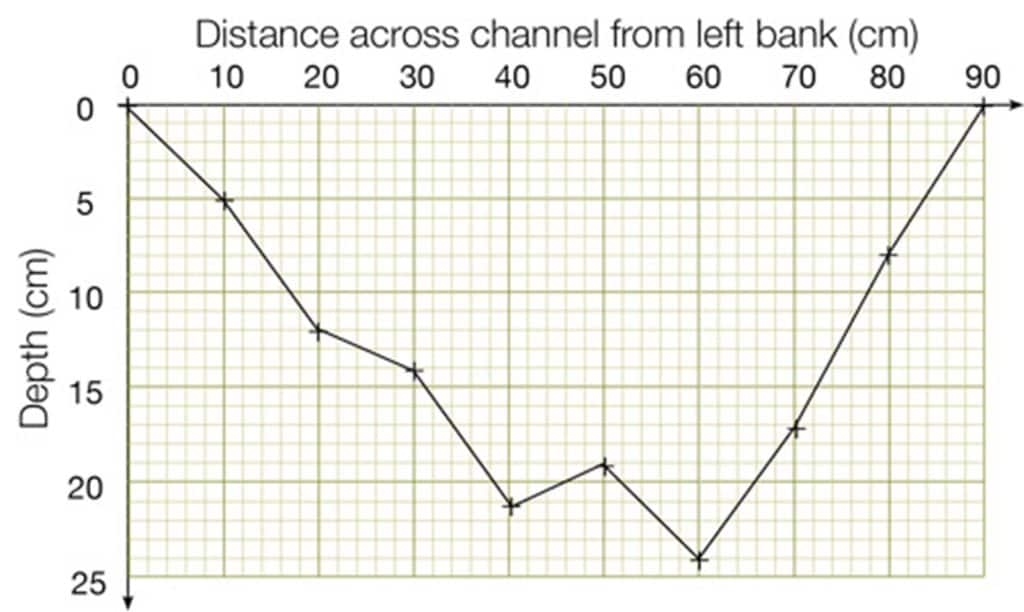### Data

Quantitative data records quantities (e.g. numbers, sizes, frequencies).

Qualitative data records subjective qualities (e.g. opinions and attitudes).

Discrete data can only take certain values (e.g. whole numbers)

Continuous data can take any value (e.g. length, width, time)

### Justifying your choice of data presentation

Your findings can be presented with a range of graphical and mapping techniques. Each form of data presentation needs to be justified. Here is an example.

### Bar charts

Bar charts are used to show the numbers of things (or frequency) in several categories

• Plot the categories on the x-axis
• Leave gaps between the bars as data are not continuous (called discrete data)

### Divided bar charts

Divided bar charts are used to show the frequency in several categories, like ordinary bar charts. It is a type of compound bar chart. But unlike ordinary bar charts, each category is subdivided.

• Plot the categories on the x-axis
• Leave gaps between the bars as data are discrete data
• Use colours or shading to show the subdivided categories, and include a key

### Percentage bar charts

Percentage bar charts are used to show the percentage of each subdivision in several categories. It is a type of compound bar chart.

• Plot the categories on the x-axis
• Leave gaps between the bars as data are discrete data
• Use colours or shading to show the subdivided categories, and include a key
• Each bar should be the same height (i.e. 100%)Example of a percentage bar chart, using the same data as the divided bar chart above.

### Histograms

Histograms are used to show the numbers of things (or frequency) along a continuous scale

• Plot the sliding scale on the x-axis
• Do not leave gaps between the bars as data are continuous data

### Pie charts

A pie chart is a circle divided into sectors. Each sector represents a percentage.

• Sectors can be shaded or coloured, and need labels or a key
• Multiple pie charts can be used where the size of each circle shows ratio.

### Line graphs

• Both axes are numerical and continuous i.e. each data point could be any value along the scale.
• If time is one of the variables, always plot it on the x-axis.Example of a line graph: both variables are continuous – each data point could be any value along the scale.A river channel profile: a special and unusual line graph.   Because we are showing the shape of the cross section we can join the points up despite it not being continous data as we are using the measurements to predict the truth.

### Scatter graphs

• This needs one independent variable (on x-axis) and one dependent variable (on y-axis).
• Both axes must show ordinal data.
• Do not join up each point: use a line of best fit instead, if appropriate.

## Secondary and Further Education Courses

Set your students up for success with our secondary school trips and courses. Offering excellent first hand experiences for your students, all linked to the curriculum.

## Group Leader and Teacher Training

Centre-based and digital courses for teachers

## Experiences for Young People

Do you enjoy the natural world and being outdoors? Opportunities for Young People aged 16-25.

## Digital Hub Plus

Subscribe/login to our package of teaching videos and resources